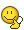BrainDen.com - Brain Teasers

## Question

The diameter of an arbitrary closed region is the greatest distance between any two of its points.

Can every region in the plane of diameter 1 fit inside a circle of diameter 2?

Edit:

It can be shown that among all closed regions of diameter 1 in the plane, the circular disk has the greatest area.

Does it follow that every region of diameter 1 will fit inside a circle of diameter 1?

Edited by bonanova
Fixing an obvious error

## Recommended Posts

• 0

...that it does not follow that every region of diameter 1 will fit inside a circle of diamter 1. The diameter is defined as the greatest distance between any two points on the boundary of a closed figure. For a polygon, it can be defined as the the greatest distance between any two vertices. Let this figure be an equilateral triangle. The diameter then is congruent and equal to any of the sides of the triangle. The altitude of this equilateral triangle is √3/2 (= cos(30°) ≈ 0.866), which is greater than the radius of the circle (r =

½), thus would extend outside the circle given that the triangle's base would need be placed congruent to the circle's diameter for the base to fit within the circle.
Edited by DejMar

##### Share on other sites
• 0

Given definition of diameter of region directly implies, that any disk of radius 1 (diameter 2) and center in region R will contain the whole R, provided diameter of R <=1. Am I missing something?

##### Share on other sites
• 0

Given definition of diameter of region directly implies, that any disk of radius 1 (diameter 2) and center in region R will contain the whole R, provided diameter of R <=1. Am I missing something?

Nope. I hit the Post button before proofreading the text.See amended OP.

## Join the conversation

You can post now and register later. If you have an account, sign in now to post with your account.×   Pasted as rich text.   Paste as plain text instead

Only 75 emoji are allowed.

×   Your previous content has been restored.   Clear editor

×   You cannot paste images directly. Upload or insert images from URL.

×